# LMS滤波结构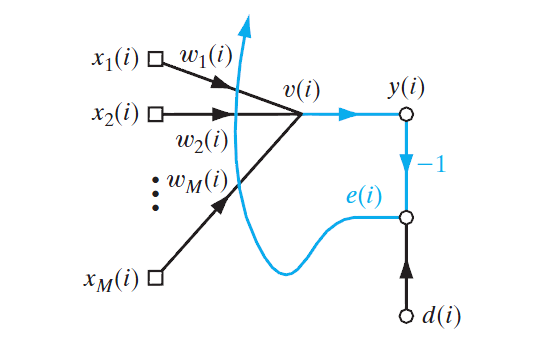y(i)=w1(i)x1(i)+w2(i)x2(i)+...+wM(i)xM(i)=k=1Mwk(i)xk(i)

y(i)=x(i)Tw(i)

w(i)$\textbf{w}(i)$即权值向量[w1(i),w2(i),...,wM(i)]T$[w_1(i),w_2(i),...,w_M(i)]^T$i$i$表示迭代次数。

e(i)=d(i)y(i)

# 无约束最优化问题

LMS算法的目标就是找到一组权值向量，使其输出响应跟期望响应最接近。

E(w)E(w)

[Ew1,Ew2,...,EwM]T=0

# 最速下降法

g=E(w)=Ew

w(i+1)=w(i)ηg(i)

η$\eta$为一个标量，称为步长或学习率参数，可以理解为沿着梯度方向走的一步的大小。

E(w)=12i=1N(diyi)2=12i=1N(diwTxi)2

g=(12i=1N(diwTxi)2)/w=i=1Neixi

w(i+1)=w(i)ηg(i)=w(i)+ηi=1Neixi

N为样本数量。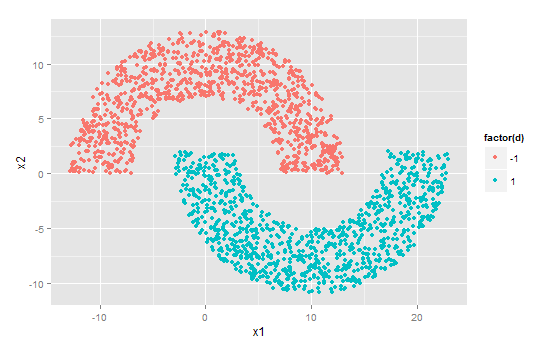> X
x1         x2
[1,]   5.394098 11.3201659
[2,]  -6.590109  4.3553063
[3,]   7.481122  4.3304918
[4,]  -5.727646  8.1037834
[5,]  -5.526536  6.9770548
[6,]   1.440511  1.5264444
[7,]  14.089176 -7.2411777
[8,]   3.846768 -1.9579111
[9,]   6.874768 -4.6271839
[10,] -10.922336  2.9085794
...

R中%*%为矩阵相乘符号，t()为矩阵转置。

# X为点的坐标数据集，d为各点的正确分类，即期望响应，值为-1和1。

W = c(0,0) #初始化权值向量
eta = 1e-6 #学习率参数
n = 50       #迭代次数

MSE = c()    #初始化均方差数组

for(i in 1:n){
y = X %*% W
e = d - y          #计算分类误差
MSE[i] = mean(e**2) #记录每一步的均方差

W = W + eta * t(X) %*% e # 修正权值
}

plot(MSE,type='l',xlab='iteration') #绘制均方差变化曲线

y = sign(X %*% W)
qplot(x1,x2,color=factor(y)) #绘制分类结果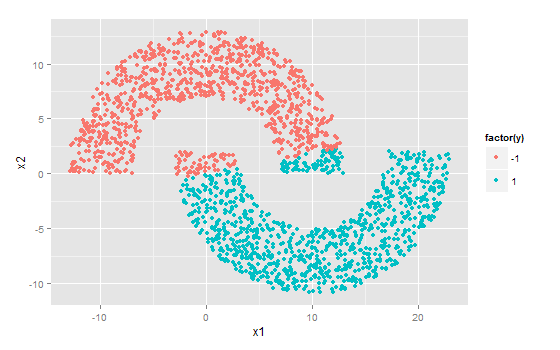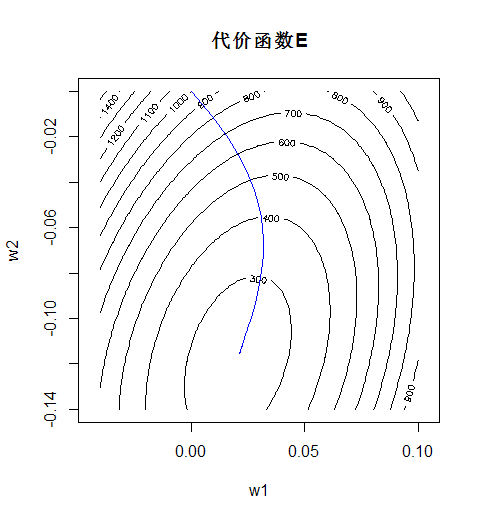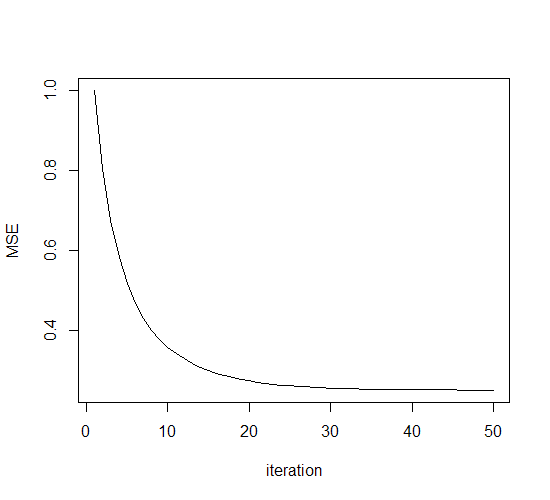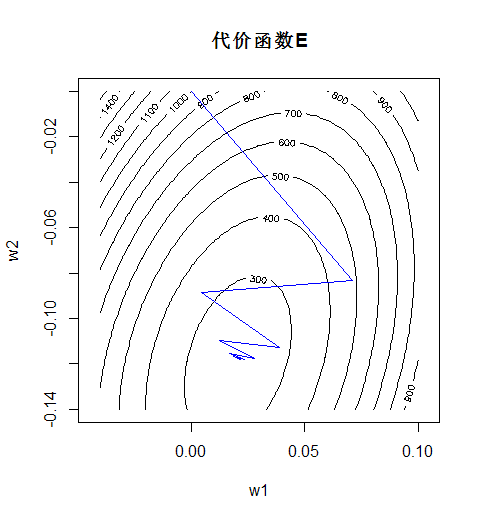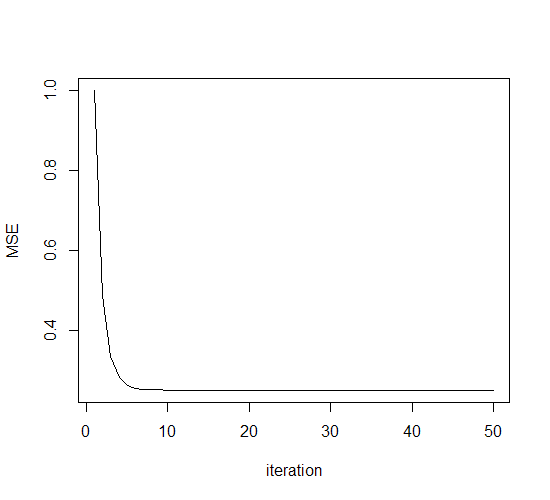# 牛顿法

ΔE(w)=E(w(i)+Δw)E(w(i))=gT(i)Δw+12ΔwTH(i)Δw

H=2Ew212Ew2w12EwMw12Ew1w22Ew222EwMw22Ew1wM2Ew2wM2Ew2M

g(i)+H(i)Δw=0

E(w)=12i=1N(diyi)2=12i=1N(diwTxi)2

hij=s=1Nxi(s)xj(s)

H=XTX

H = t(X) %*% X #计算Hessian矩阵

W = c(0,0) #初始化权值向量
n=50

for(i in 1:n){
y = X %*% W
e = d - y

g = - t(X) %*% e
W = W - solve(H) %*% g #按照公式修正权值
}

R中函数solve()可以求解矩阵的逆。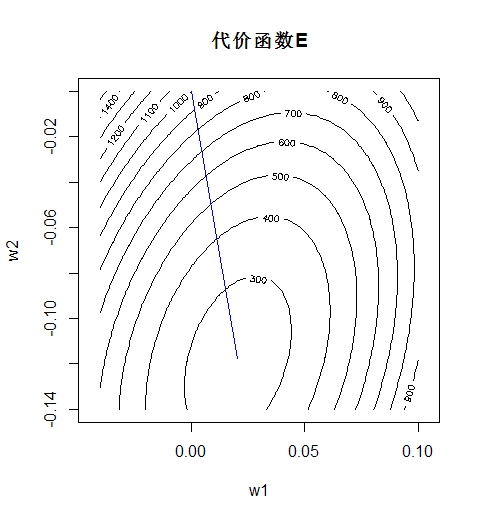# 高斯-牛顿法

E(w)=12i=1N(diyi)2=12i=1Ne2i

ei(w)=ei(w(n))+[eiw]T(ww(n))

e(w)=e(w(n))+J(n)(ww(n))

J$\textbf J$为Jacobi矩阵：

J=e1w1e2w1eNw1e1w2e2w2eNw2e1wMe2wMeNwM

E(w)=12e(w)2=12e(w(n))2+e(w(n))TJ(n)(ww(n))+12(ww(n))TJ(n)TJ(n)(ww(n))

JT(n)e(n)+JT(n)J(n)(ww(n))=0

w(n+1)=w(n)(JT(n)J(n))1JTe(n)

w(n+1)=w(n)(JT(n)J(n)+δI)1JTe(n)

## 维纳滤波器

J=[x1,x2,...,xN]T=X

X$\textbf X$就是样本矩阵。

w(n+1)=w(n)+(XT(n)X(n))1XT(d(n)Xw(n))

w(n+1)=(XT(n)X(n))1XT(n)d(n)

w(n+1)=X+d(n)

R语言中用行代码即可算得权值向量：

W = solve(t(X) %*% X) %*% t(X) %*% d

# 最小均方算法

E(w)=12(diyi)2=12e2i

Ew(n)=x(n)e(n)

w(n+1)=w(n)+ηx(n)e(n)

η$\eta$同上为学习率参数。

R代码实现如下：

W = c(0,0) #初始化权值
eta = 1e-4 #学习率参数
n = 5 #进行5轮迭代

MSE = c() #均方根误差记录向量

for(t in 1:n){
for(i in 1:N){
e = d[i]-X[i,] %*% W
W = W + e*X[i,] #修正权值

E = d - X %*% W
MSE = append(MSE,mean(E**2)) #计算并记录均方差
}
}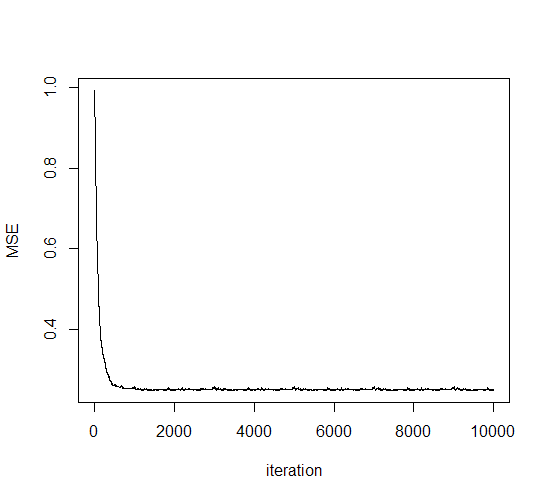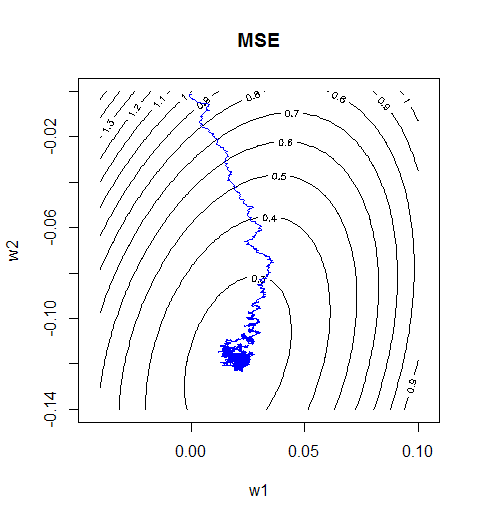# 学习率退火方案

η(n)=η01+n/τ

07-231324

#### 最小均方算法LMS总结

07-23654

#### 最小均方算法（LMS）04-243610

#### 机器学习（三）——最小均方算法（LMS algorithm）

11-261477

#### 最小均方法（LMS）

07-293万+

#### LMS算法概述

03-049401

#### 什么是LMS算法（Least mean square）

10-052万+

#### 自适应滤波-----LMS（Least Mean Square）算法

07-264万+

#### 最小均方算法（LMS）的原理

03-012万+

#### 将一个接口响应时间从2s优化到 200ms以内的一个案例

05-312万+

#### 自适应滤波之最小均方（LMS）算法以及matlab实现

03-227万+

#### 男生更看重女生的身材脸蛋，还是思想？

05-078730

#### 自适应滤波器：最小均方误差(LMS)滤波器

12-122万+

#### 训练数据常用算法之Levenberg–Marquardt（LM）

07-09801

#### LMS算法

12-192106

#### 神经网络学习笔记（九）:多层感知机（上）

03-281925

#### 【机器学习算法笔记】2. 学习算法与最小均方算法（LMS）

07-1022万+

#### Java 最常见的 200+ 面试题：面试必备©️2020 CSDN 皮肤主题: 大白 设计师: CSDN官方博客点击重新获取扫码支付1.余额是钱包充值的虚拟货币，按照1:1的比例进行支付金额的抵扣。
2.余额无法直接购买下载，可以购买VIP、C币套餐、付费专栏及课程。余额充值